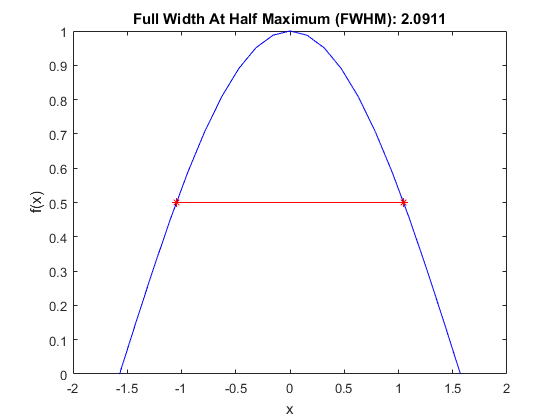fwhm

Compute the full width at half maximum.

Syntax

fwhm_val = fwhm(f, x)
fwhm_val = fwhm(f, x, plot_fwhm)

Description

fwhm calculates the Full Width at Half Maximum (FWHM) of a positive 1D input function f(x) with spacing given by x.

For example, running the code

x = -pi/2:pi/20:pi/2;
f = cos(x);
fwhm(f, x, true);

produces the outputInputs

 f f(x) x x data or dx

Optional Inputs

 plot_fwhm Boolean controlling whether a plot of the function and FWHM is produced

Outputs

 fwhm_val FWHM of f(x)